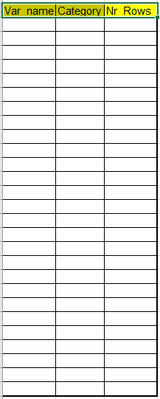## Freq table for all variables that have less then 100 categories

Hello friends,

I have a very big data set with 250 million rows and 20 variables.

I would like to create a data set with the following information:

For each variable that have less then 100 categories I want to see how many rows are in each category (also show category of missing value)

The wanted data set will have the following structure:May anyone show a nice code that do it?

Please remember that my raw data set  is very big as I mentioned before

1 ACCEPTED SOLUTION

Accepted Solutions

## Re: Freq table for all variables that have less then 100 categories

A place to start:

```ods select nlevels;
proc freq data=yourdatasetnamegoeshere nlevels;
ods output nlevels=levelsdata;
run;```

This will create a data set with the total number of levels, the number of missing levels (different missing values) and number of nonmissing levels.

I suspect that what you mean by "less than 100 categories" is looking for the NNonMissLevels variable in the created set unless "(also show category of missing value" means count the missing as well.

You can use the information from the Nlevels data to select variables as one of the variables in the table is TableVar that will have, with this example, the name of the variable. So filter where whichever definition of category fits to get the names of the variables.

Here's an example using the SASHELP.Class data set you should have to test code:

```ods select nlevels;
proc freq data=sashelp.class nlevels;
ods output nlevels=levelsdata;
run;

proc sql noprint;
select tablevar into :varlist separated by ' '
from levelsdata
where nlevels le 100
;
quit;

proc freq data=sashelp.class;
ods output onewayfreqs= rowcount;
tables &varlist.;
run;

data want;
set rowcount (rename=( frequency = NrRows));
name= scan(table,2);
category = left(coalescec( of f_:));
keep name category nrrows;
run;```

Caveat: the Category variable may need to have a length defined prior to use if YOUR values exceed 200 characters in length.

The  Proc SQL is one way to get a list of values from a data set into a macro variable that can be used elsewhere, such as a Tables statement in the example.

4 REPLIES 4

## Re: Freq table for all variables that have less then 100 categories

PROC FREQ with the LIST option ought to do this.

The fact that it is a huge table doesn't change the fact that you will have to run PROC FREQ to get that information. (Or possibly use hash objects)

--
Paige Miller

## Re: Freq table for all variables that have less then 100 categories

MAy you please show the code on sashelp.class for example? May you please get list on variables with not more then 100 categories and then perorm the required fruency only on them and put the information in one data set?

## Re: Freq table for all variables that have less then 100 categories

Surely you can write PROC FREQ code yourself

--
Paige Miller

## Re: Freq table for all variables that have less then 100 categories

A place to start:

```ods select nlevels;
proc freq data=yourdatasetnamegoeshere nlevels;
ods output nlevels=levelsdata;
run;```

This will create a data set with the total number of levels, the number of missing levels (different missing values) and number of nonmissing levels.

I suspect that what you mean by "less than 100 categories" is looking for the NNonMissLevels variable in the created set unless "(also show category of missing value" means count the missing as well.

You can use the information from the Nlevels data to select variables as one of the variables in the table is TableVar that will have, with this example, the name of the variable. So filter where whichever definition of category fits to get the names of the variables.

Here's an example using the SASHELP.Class data set you should have to test code:

```ods select nlevels;
proc freq data=sashelp.class nlevels;
ods output nlevels=levelsdata;
run;

proc sql noprint;
select tablevar into :varlist separated by ' '
from levelsdata
where nlevels le 100
;
quit;

proc freq data=sashelp.class;
ods output onewayfreqs= rowcount;
tables &varlist.;
run;

data want;
set rowcount (rename=( frequency = NrRows));
name= scan(table,2);
category = left(coalescec( of f_:));
keep name category nrrows;
run;```

Caveat: the Category variable may need to have a length defined prior to use if YOUR values exceed 200 characters in length.

The  Proc SQL is one way to get a list of values from a data set into a macro variable that can be used elsewhere, such as a Tables statement in the example.

Discussion stats
• 4 replies
• 152 views
• 1 like
• 3 in conversation